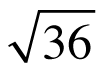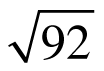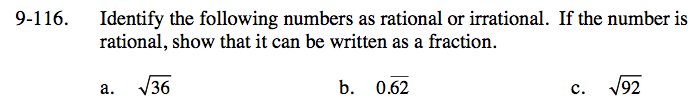Home > CC3 > Chapter 9 > Lesson 9.2.4 > Problem9-116

9-116.
1. Identify the following numbers as rational or irrational. If the number is rational, show that it can be written as a fraction. Homework Help ✎

1.2. 0.62

3.Is there a whole number that can be squared to get 36?

$\sqrt{36} = 6 = \frac{6}{1}$

Is this number rational?

Use the method you learned earlier to convert this number to a fraction.

.62 has two repeating digits, so you put them over 99.

$\frac{62}{99}$

Is this number rational?

Is the square root of 92 a whole number, a terminating decimal, or a repeating decimal?